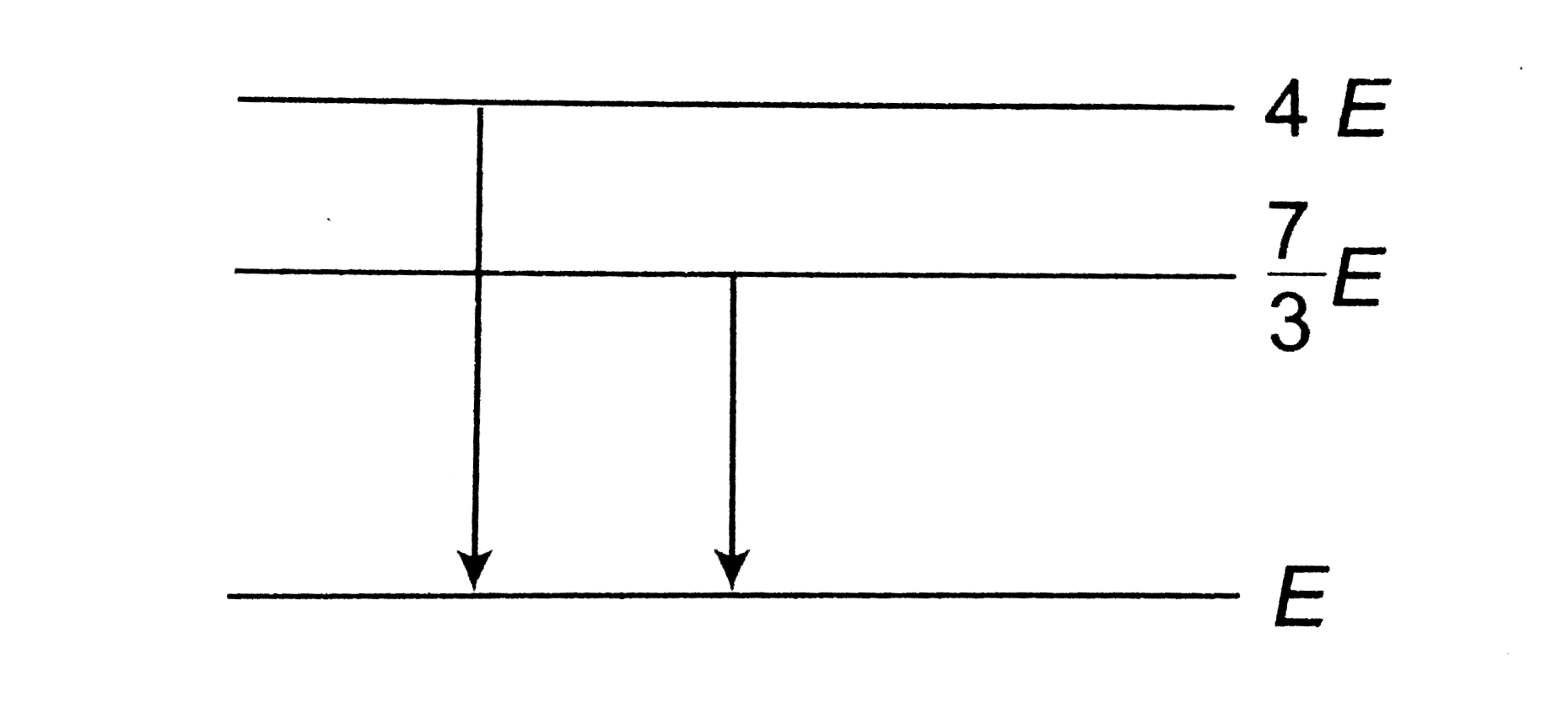# The following diagram indicates the energy levels of a certain atom when the system moves from 4E level to E. A photon of wavelength lambda_(1)

23 views
in Physics
The following diagram indicates the energy levels of a certain atom when the system moves from 4E level to E. A photon of wavelength lambda_(1) is emitted. The wavelength of photon produced during its transition from (7)/(3)E level to E is lambda_(2). the ratio (lambda_(1))/(lambda_(2)) will beby (24.7k points)